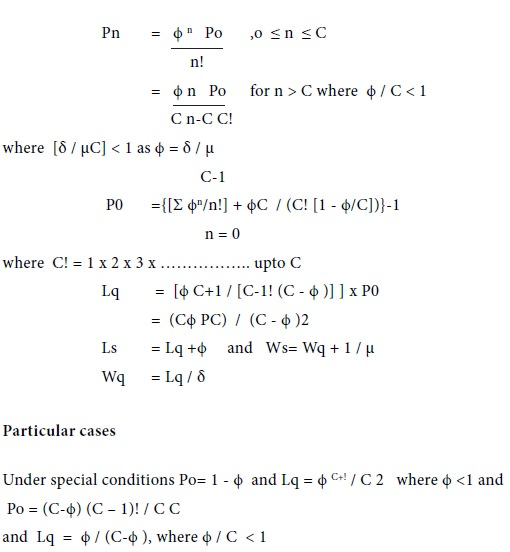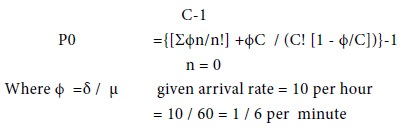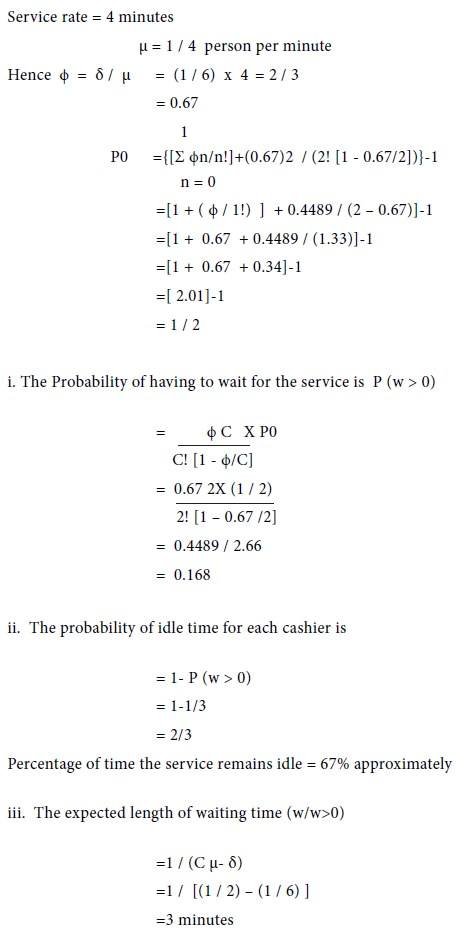Home | ARTS | Operations Management | Model 2 : (M/M/C) : (GD/ ∞/ ∞ ) Model - Types of Waiting Line Models - Queueing Theory

# Model 2 : (M/M/C) : (GD/ ∞/ ∞ ) Model - Types of Waiting Line Models - Queueing Theory

Posted On :  25.06.2018 02:43 am

The following assumptions are made for this model

Model 2 : (M/M/C) : (GD/ / ) Model

The following assumptions are made for this model:

The arrival rate follows Poisson distribution

The service rate follows Poisson distribution

The number of servers is C

The service discipline is general discipline

The maximum number of customers allowed in the system is infinite

With these assumptions, the steady state equation for the probability of having n customers in the system is given byExample 1

At a Toll Gate, vehicles arrive at the rate of 24 per hour and the arrival rate follows Poisson distribution. The time to collect a toll and permitting the vehicle to pass follows exponential distribution and the passing rate is 18 vehicles per hour. There are 4 passing counters. Determine the following:

1.  Po and P3

2.  Lq, Ls, Wq and Ws

Solution

The arrival rate δ= 24 per hour.

The passing rate µ = 18 Per hour.

No. of passing counters C=4.Example 2

In a bank, there are two cashiers in the cash counters. The service time for each customer is exponential with mean 4 minutes and the arrival rate of the customers is 10 per hour and the arrival of the customers follows Poisson distribution. Determine the following:

1.      The probability of having to wait for service

2.      The expected percentage of idle time for each cashier

3.      Whenever a customer has to wait, how much time he is expected to wait in the Bank?

SolutionTags : Operations Management - Game Theory, Goal Programming & Queuing Theory
Last 30 days 291 views